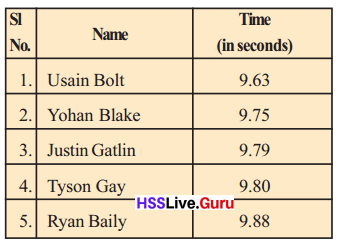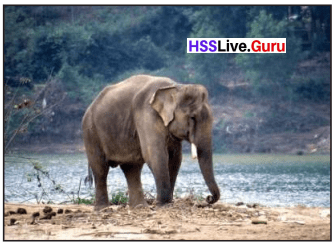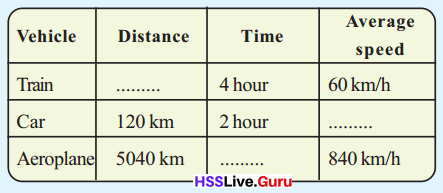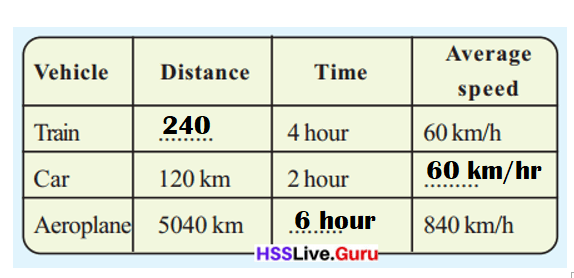# Kerala Syllabus 7th Standard Maths Solutions Chapter 7 Speed Math

You can Download Speed Math Questions and Answers, Activity, Notes, Kerala Syllabus 7th Standard Maths Solutions Chapter 7 help you to revise complete Syllabus and score more marks in your examinations.

## Kerala State Syllabus 7th Standard Maths Solutions Chapter 7 Speed Math

### Speed Math Text Book Questions and Answers

Olympics 2012 Textbook Page No. 90

Look at the table showing the first five in men’s 100 metres sprint in the London OlympicsHow much time do you take to run 100 metres?The time taken by me can be calculated by the following formula:
Speed = Distance ÷ Time.

Explanation:
Time taken to run in 100 meters sprint in the London Olympics:
speed = distance ÷ time.Rotating earth

Do we ever remain still? The Earth which carries us all, turns all the time, spinning on its own and going round the Sun. It spins at about 1700 km/h and revolves round the Sun at about 100000 km/h.Value of time Textbook Page No. 93

The smallest unit of time we usually use is a second. Sometimes we need to use smaller units such as a microsecond or a nanosecond. A microsecond is a millionth of a second. A nanosecond is a thousandth of a microsecond.
Do you know by what part of a second did P.T. Usha lose an Olympic medal?
By 1/100th part of a second P.T. Usha lose an Olympic medal.

Explanation:
At the Games, she clocked 56.81 s in the heats and 55.54 s in the semi-final, setting a new Commonwealth record as she entered the final. At the final, she came fourth, at 55.42 seconds, falling behind the eventual bronze medalist by 1/100th of a second.Look at the average speeds of some animals:Over speeding

How much does a car doing 90 km/h move in a minute?
$$\frac{90}{60}$$ = $$\frac{3}{2}$$ = 1$$\frac{1}{2}$$ km
And in one second?
1$$\frac{1}{2}$$ kilometers means 1500 metres.
$$\frac{1500}{60}$$ = $$\frac{75}{3}$$ = 25 metres
So what if the driver is one second late in applying the brakes? The vehicle would have moved 25 metres ahead.
If the driver is one second late in applying the brakes, the vehicle would have moved 25 metres ahead in a slower speed.

Explanation:
If the driver is one second late in applying the brakes,the vehicle would have moved 25 metres ahead.
=> This shows that force can make a moving object go slower.Road accidents Textbook Page No. 96

We read about traffic accidents every day in the papers. The main causes are speeding and carelessness. How many lives are lost on our roads everyday!
There is a law that heavy vehicles should in stall a speed-lock”. With this device, they can not go beyond a certain speed.
If each of us obey the traffic rules, we can reduce the number of accidents.

Who’s the fastest? Textbook Page No. 90

“We have to find the fastest runner in the school. How do we do it?” The teacher asked.
“Let everyone run 100 metres”, Raji said.
Reghu had another idea: “How about everyone running for 1 minute?”
They all went to the ground.
And all ran 100 metres.
These were the best four:Who won the race?
Is it easy to conduct a race as Reghu suggested?
Shyam won the race.
No, its not easy to conduct a race as Reghu suggested as its limited by time and distance and strength of students is more.

Explanation:
According to the speed chart of the students. Shyam has won the race as time taken by him is comparatively less than other students.
Its not easy to conduct a race as Reghu suggested because time limitation is high n distance to run is longer and speed of the student has to be more.

Sports meet

Reghu and friends went in a bus to the sports meet at Kozhikode. They started at 7 in the morning and reached there at 10, travelling 150 kilometres. Would the speed have been the same during the entire trip?
Could have been 40 kilometres the first hour, 60 kilometres the next and 50 kilometres the last hour.
It’s in such instances that we calculated the average, remember?
Here, the distance travelled is 150 kilometres.
And how much time did it take?
So, we can say they travelled $$\frac{150}{3}$$ = 50 kilometres in one hour, on average.
We can put it this way: the average speed of the bus was 50 kilometres per hour,
This we write 50 km/h.

Average speed Textbook Page No. 91

Celina and Beena went to Kozhikode for the State Arts Festival. Celina travelled in a jeep, covering 90 kilometres in 2 hours; Beena came in a car, travelling 150 kilometres in 3 hours. Which vehicle is faster?
The jeep travelled 90 kilometres, right?
And took 2 hours for the trip.
So what is its average speed?
$$\frac{90}{2}$$ = 45 km/h
The average speed of the car can also be computed like this.
Which is greater?
Now try to solve these problems:

• Sudheer travelled in a train, covering 240 kilometres in 3 hours to reach Thiruvananthapuram. Ramesh came in another train, travelling 120 kilometres in 2 hours. Which train is faster? How much faster?
Sudheer train travelled faster by 20 km/hr than Ramesh train.

Explanation:
Distance travelled by Sudheer in a train = 240 km.
Time taken by Sudheer = 3 hours.
Distance travelled by Ramesh in a train = 120 km.
Time taken by Ramesh = 2 hours.
Speed taken by Sudheer train = Distance travelled by Sudheer in a train ÷ Time taken by Sudheer
= 240 ÷ 3
= 80 km/ hr.
Speed taken by Ramesh train = Distance travelled by Ramesh in a train ÷ Time taken by Ramesh
= 120 ÷ 2
= 60 km/ hr.
Difference:
Speed taken by Sudheer train – Speed taken by Ramesh train
= 80 – 60
= 20 km/hr.

• A train took 4 hours and 30 minutes to cover 360 kilometers. What is its average speed?
Speed of the train = 80 km/hr.

Explanation:
Time taken by a train = 4 hours and 30 minutes = 4$$\frac{1}{2}$$
Distance travelled = 360 km.
Speed of the train = Distance travelled ÷ Time taken by a train
= 360 ÷ 4$$\frac{1}{2}$$
=  360 ÷ [(4× 2) + 1) ÷ 2]
= 360 ÷ $$\frac{9}{2}$$
= (360 × 2) ÷ 9
= 720 ÷ 9
= 80 km/hr.

Let’s look at another problem.
If the average speed of a bus is 52 km/h, how much does it travel in 6 hours?
Since it travels 52 kilometres each hour on average, in 6 hours it travels
52 × 6 = 312 km
How long does it take to travel 520 kilometres at this speed?

• The table below gives some details of Joy’s trip. Fill in the missing entries:Missing entries details of Joy’s trip:Explanation:
Speed of the train = Distance travelled ÷ Time taken by a train
=> 60 km/hr = Distance travelled ÷ 4 hrs
=> 60 × 4 = Distance travelled
=> 240 km = Distance travelled.

Speed of the car = Distance travelled ÷ Time taken by a car
=> Speed of the car = 120km ÷ 2 hrs
=> Speed of the car = 60 km/ hr.

Speed of the Aero plane = Distance travelled ÷ Time taken by a Aero plane
=> 840 km/hr = 5040 km ÷ Time taken by a Aero plane
=> Time taken by a Aero plane × 840 = 5040
=> Time taken by a Aero plane = 5040 ÷ 840
=>  Time taken by a Aero plane = 6 hrs.

• Shyama’s exam starts at 2 o’ Hoot To reach the place, she has to travel 50 kilometres by bus and 175 kilometres by train. Average speed of bus journey is 20 km/h and average speed of train journey is 50 km/h. To reach there 1 hour before the exam, when should Shyama leave home?
Time Shyama leave home = 7 AM.

Explanation:
Time of Shyama’s exam starts = 2 pm.
Distance travelled by bus = 50 km.
Average speed of bus = 20 km/ hr.
Distance travelled by train = 175 km.
Average speed of train = 50 km/ hr.
To reach there 1 hour before the exam.
Time taken by bus she travels = Distance travelled by bus ÷ Average speed of bus
=> Time taken by bus she travels = 50 ÷ 20
=> Time taken by bus she travels = 2.5 hrs = 2 hour 50minutes.
Time taken by train she travels = Distance travelled by train ÷ Average speed of train
=> Time taken by train she travels = 175 ÷ 50
=> Time taken by train she travels = 3.5 hrs = 3 hour 50 minutes.
=> Time Shyama has to travel = Time taken by bus she travels  +  Time taken by train she travels
=> 3.50 + 2.50
=> 6 hrs.
Time Shyama leave home = 2pm – Time Shyama has to travel
=>2 pm – 6 hrs – 1 hr
=> 7 AM.Saving time

A bus leaves Ernakulam at 6 in the morning and reaches Thiruvananthapuram at 12 noon, running 40 km/h on average. To reach one hour earlier, by how much should the average speed be increased?
What is the total distance covered?
If the journey time is to be reduced by 1 hour, what should be the time for the trip?
What should be the average speed to reach an hour earlier?
The total distance covered = 240 km.
The average speed to reach an hour earlier is 48 km/hr.

Explanation:
A bus leaves Ernakulam at 6 in the morning and reaches Thiruvananthapuram at 12 noon.
Average speed of the bus = 40 km/h.
Time taken by bus to travel from Ernakulam to Thiruvananthapuram  = 12 noon – 6am = 6 hours.
Speed = Distance ÷ Time.
=> 40 = Distance ÷ 6
=> 40 × 6 = Distance
=> 240 km = Distance

If the journey time is to be reduced by 1 hour,
Time taken = Time taken by bus to travel from Ernakulam to Thiruvananthapuram  – 1 hr
=> 6 hr –  1hr
=> 5 hours.
Speed = Distance ÷ Time.
=> Speed = 240 ÷ 5
=> Speed = 48 km/hr.

Railway station Textbook Page No. 93

Abu boarded a bus at 7 in the morning. Usually the bus travels 30 km/h on average, to reach the railway station at 11. But since it was raining, the bus could run only 20 km/h on average. Abu got down from the bus at 9, caught a car and reached the station at 11 itself. What was the average speed of the car?
What is the distance to the railway station?
How far did the bus travel in the first 2 hours?
So how many kilometres did the car travel?
How much time did it take?
Distance to the railway station = 120 km.
40 kms the car travelled.
Distance travelled in 2 hours by bus = 60 km.
It took 2hrs of time by car.

Explanation:
Speed of bus = 30 km/h
Time of Abu boarded the Bus = 7 AM.
Time to reach the railway station = 11 AM.
Time taken = 11 – 7 = 4 hrs.
Due to raining, the speed of bus = 20km/ hr.
Time Abu got down the bus = 9 AM.
Time Abu reached the station = 11AM.
Speed = Distance ÷ Time.
=> 30 = Distance ÷ 4
=> 30 × 4 = Distance
=> 120 kms = Distance.
Distance travelled in 2 hours:
Speed = Distance ÷ Time.
=> 30 = Distance ÷ 2
=> 30 × 2 = Distance
=> 60 kms = Distance.
Due to raining:
Speed = Distance ÷ Time.
=> 20 = Distance  ÷ 2
=> 20 × 2 = Distance
=> 40 km = Distance.

Average of speeds and average speed

A vehicle did the first 120 kilometres of a trip at an average speed of 30 km/h and the next 120 kilometres at 20 km/h. What is the average speed of the entire trip?
The average of the two speeds is
$$\frac{30+20}{2}$$ = 25 km/h
Is this what we want?
What is the correct reasoning?

To compute the average speed, we must divide the total distance by the total time. Time to travel 120 kilometres at
an average speed of 30 km/h is
$$\frac{120}{30}$$ = 4 hours
Time to travel 120 kilometres at an average speed of
$$\frac{120}{20}$$ = 6 hours
Total time of travel 4 + 6 = 10 hours
Total distance travelled = 240 km
Average speed = 24 km/h

Train and bus Textbook Page No. 94

Rahim travelled 350 kilometres in a train and 150 kilometres in a bus. The average speed of the train was 70 km/h. The bus trip lasted 5 hours. What is the average speed of the entire trip?
Average speed of the entire trip = 50km/hr.

Explanation:
Distance Rahim travelled by train  = 350 km.
Distance Rahim travelled by bus = 150 km.
Average speed of the train = 70 km/h.
Time Rahim travelled by bus = 5 hours.
Average Speed of the bus = Distance Rahim travelled by bus ÷ Time Rahim travelled by bus
=> Average Speed of the bus = 150 ÷ 5
=> Speed of the bus = 30 km/hr.
Average speed of the entire trip = (Average speed of the train + Average Speed of the bus) ÷ 2
= (70 + 30) ÷ 2
= 100 ÷ 2
= 50km/hr.

To Ratnagiri

Ratnagiri is 360 kilometres from Pavizhamala. Gopika’s family travelled in a car, at an average speed of 60 km/h. They managed only 40 km/h during the return trip. What is the average speed of the entire trip?
Suppose we take the distance as 180 kilometres in this problem.
Does it change the average speed for the entire journey?
No, it does not change the average speed for the entire journey because speed is not dependent of distance travelled.

Explanation:
Distance from Pavizhamala to Ratnagiri = 360 km.
Average speed Gopika’s family travelled in a car = 60 km/h.
Average speed they managed during the return trip = 40 km/h.
Average speed of the entire trip = (Average speed Gopika’s family travelled in a car + Average speed they managed during the return trip) ÷ 2
= (60 + 40) ÷ 2
= 100 ÷ 2
= 50 km/hr.
Suppose, Distance travelled = 180 km.
Average speed Gopika’s family travelled in a car = 60 km/h.

Unknown distance

Babu went to Mananthavady by bus to see his friend. The average speed of the bus was 40 km/h. He came back in a car, at an average speed of 60 km/h. What is the average speed of the entire trip?
To find this, we have to divide the total distance by the total time. But we don’t know the distance.
We have seen in an earlier problem the average speed would be the same, whatever be the total distance.
Let’s take the distance one way as 120 kilometres. Total distance is then 240 kilometres
Time for the onward trip = $$\frac{120}{40}$$ = 3 hours
Time for the return trip = 2 hours
So, the average speed of the entire trip = 48 km/h
What if we take the one way distance as 240 kilometres?
Can’t we find the time of this trip?
The time of this trip = 6 hours.

Explanation:
Average speed of the bus = 40 km/h.
He came back in a car,
=>Average speed of car = 60 km/h.
Average speed of the entire trip = (Average speed of the bus + Average speed of car) ÷ 2
= (40 + 60) ÷ 2
=> 100 ÷ 2
=> 50 km/hr.
Distance travelled one trip = 240 km.
Average speed of the bus = 40 km/h.
Speed = Distance ÷ Time
=> 40 = 240 ÷ Time
=> Time = 240 ÷ 40
=> Time = 6 hours.

Johny’s journey Textbook Page No. 95

• Johny went to his uncle’s house, riding his bicycle at 15 km/h and came back at 10 km/h. What’s his average speed for the whole journey?
Average speed for the whole journey = 12.5 km/ hr.

Explanation:
Speed of his bicycle = 15 km/h
Speed of his bicycle while coming back = 10 km/h
Average speed for the whole journey = (Speed of his bicycle + Speed of his bicycle while coming back) ÷ 2
= (15 + 10) ÷ 2
= 25 ÷ 2
= 12.5 km/ hr.

In seconds

A vehicle travels at an average speed of 72 km/h. How much will it move in one second?
One hour means 60 minutes; and a kilometre is 1000 metres.
Thus in 60 minutes, this vehicle will travel 72000 metres.
So, in one minute, it would move $$\frac{72000}{60}$$ = 1200 metres.
Which means that in one second it would move $$\frac{1200}{60}$$ = 20 meters.
We can say that the average speed of the car is 20 meters per second, written 20 m/s.
Compute the speed per hour of a vehicle doing 15 m/s.
Speed = 54000 m/ hr or 54 km/hr.

Explanation:
A vehicle travels at an average speed = 72 km/h.
Conversion:
1 hour = 3600 seconds.
A vehicle moves per second = A vehicle travels at an average speed ÷ 3600
=> 72 ÷ 3600
=> 0.02 km/ second.
Speed per hour of a vehicle doing 15 m/s.
=> Speed = 15 m/s
=> 15 × 3600
=> 54000 m/ hr.
Conversion:
1 km = 1000 m.
=> 54000m ÷ 1000m = 54 km.

Now try these problems.

• A train travels at an average speed of 36 km/h. How far will it go in 3 minutes?
1.8 km the train goes in 3 minutes.

Explanation:
Average speed of a train travels  = 36 km/h
Time = 3 minutes.
Speed = Distance ÷ Time
Conversion:
1 hour = 60 minutes
=> 36 ÷ 60 = 0.6 km/min
=> 0.6 × 3 minutes = 1.8 km/ 3 mins

• A 180 meters long train takes 9 seconds to pass a vertical pole. What is the average speed of the train in kilometer per hour?
Speed of the train = 72 km/hour.

Explanation:
Distance of train = 180 meters.
Time taken to pass a vertical pole = 9 seconds.
Average speed of the train = Distance ÷ Time
=> Speed = 180 ÷ 9
=> Speed = 20 m/s.
Conversion:
1 hour = 3600.
=> Average speed of the train = Distance ÷ Time
=> 20 × 3600
=> 72000 m/hour.
Conversion:
1 km = 1000 m.
=> Speed = 72000 ÷ 1000
=> Speed = 72 km/hour.Let’s do it! Textbook Page No. 95

Question 1.
A car goes an average speed of 36 km/h for 15 minutes and 60 km/h for the next 15 minutes. How much distance did it cover?
Total distance covered = 1,440 km.

Explanation:
Speed of a car = 36 km/h
Time of a car = 15 minutes
Speed = Distance ÷ Time
36 = Distance ÷ 15
=> 36 × 15 = Distance
=> 540 km = Distance.
Speed of a car = 60 km/h
Time of a car = 15 minutes
Speed = Distance ÷ Time
=> 60 = Distance ÷ 15
=> 60 × 15 = Distance
=> 900 km = Distance
Total distance covered = 540 + 900 = 1,440 km

Question 2.
Ramu and Salim travelled to Thiruvananthapuram from the same place, each in his own car. Ramu did the onward journey at an average speed of 120 km/h and the return at 50 km/h. Salim did both trips at 60 km/h. Who made the total journey in less time?
Ramu made the total journey in less time.

Explanation:
Average speed of Ramu the onward journey = 120 km/h
Average speed of Ramu the return journey = 50 km/h
Average speed of Ramu of the journey = (Average speed of Ramu the onward journey + Average speed of Ramu the return journey) ÷ 2
=> (120 + 50) ÷ 2
=> 170 ÷ 2
=> 85 km/ hr.
Average speed of Salim the both journeys = 60 km/hr.

Question 3.
Two trains travelling along parallel tracks in the same direction do 50 km/h and 100 km/h on average. The faster train starts 2 hours later than the slower. After how many kilometers will the faster train catch up?
After 200 km the faster train will catch up.

Explanation:
Average speed of both Trains = 50 km/h and 100 km/h
The faster train starts 2 hours later than the slower.
=> Let first train be slower = 60 km/ hr.
=> Second train be faster = 100 km/ hr.
Speed of the first train = Distance ÷  Time
=>Let time taken by slower train to where it caught by faster one = x hour
And time taken by faster one upto where it catch = (x – 2)
=> Distance = speed × time
thus we have x × 50 = (x – 2)×100
50x = 100x- 200
50x = 200
x= 4 hours
So after 4 hours faster train catch slower one
Number of km at which it catch = 4×50 =200 km.

Question 4.
A 125 metre long train travels at 90 km/h. How much time would it take to cross a 175 meter long bridge?
12 seconds time would it take to cross a 175 meter long bridge.

Explanation:
Length of the train = 125 m.
Speed of the train = 90 km/h.
First convert 90 km/hr to m/hr.
Conversion:
1 km = 1000 m.
90 x 1000 = 90,000 m/hr.
Conversion:
1hour = 3600 seconds.
90,000 ÷ 3600 = 25m/sec.
=> the train is traveling at 25 m/sec
Distance = speed × time
=> time = Distance ÷ speed
=> time = 175/25
=> time =  7 seconds.
Time at which the front of the train will cross the 175 meters.
The train is 125 meters long, so at 25 m/sec,
=> it will take another 5 seconds (125/25) for the rear of the train to cross the 175 meter distance.
Total time taken = 7 seconds + 5 seconds
=> Total time taken = 12 seconds.